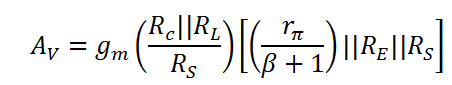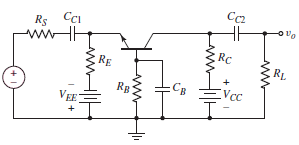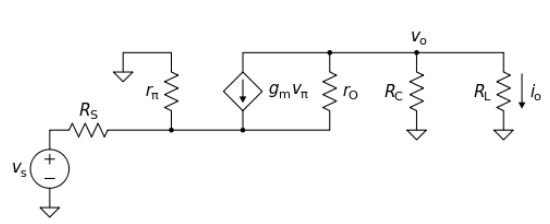# Common Base Amplifier Calculator (Small Signal Analysis)

This calculator computes the Diffusion resistance (rp), Small signal transconductance (gm) and Output Voltage gain (Av) for a common base amplifier, i.e the small signal parameters and output gain of a Self-Biased Common Base (CB) BJT Amplifier using its Hybrid Pi model. In this configuration the emitter of the BJT is the input, the collector is the output and the base is the common port, which is usually connected to ground. This configuration is equivalent to the common gate configuration in a FET transistor.

Here input resistance r0 has been assumed to be infinite.

*Temperature has been asked to calculate the thermal voltage. Please enter T=300K if nothing is given.

mA
K
K-Ohm
K-Ohm
K-Ohm
K-Ohm

#### Result

K-Ohm
mA/V
V/V